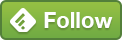## Apr 30 Excel Tricks For Digital Analysts - Part 2

In part 1 of this post, we focused mainly on text extraction [and labelling it] to identify contest posts/questions/audience tags etc. Here's a link to part 1 of the post: Excel tricks for digital analysts.

1. Combining tables where some of the columns are different.

If you downloaded post level insights [in csv] for two different time periods, there is a possibility that the number of columns might not match, especially engagement metrics for video [if the post type wasn't used in the period]. A csv Facebook post insights download could have approximately 90 columns. Therefore, finding the missing/extra columns between two csv files would be rather impractical.

How would you find this in Excel?

Power Query plug-in can help solve this problem by creating a third pivot table [combining the two csv data insights] as long as there is a primary key. In this case, this could be a post ID.

Let's say our likes and comments are in the first download and the shares for the same posts are in the second file. Both files have a post ID column where the posts are the primary key [1 -10]. This is how the files would look.

Open Query tab and add data from table.

Now click on New Source > File from the right hand corner and add the second file.

Now if you appended the first table to the second one, you would see something like this:

Once you pivot this again, you'd have your likes, comments and shares by the unique post ID.

2. Finding number of words in a post.

While number of characters in a post can be easily determined using the LEN formula, finding the number of words requires a bit of trick.

How would we do this in Excel?

Breakdown of this formula:

First part:

TRIM function removes extra spaces in a cell. This can be helpful in case of web downloads.

LEN function would then count the number of characters in the cell.

Second part:

SUBSTITUTE function removes blank spaces and then counts the number of character (after trimming the text).

The difference in LEN between the first and second part of the formula tells us how many blank spaces we have. Adding +1 to tricks Excel into giving us the number of words. To understand this a bit more. The number of words in a sentence will always be the number of spaces between words + 1.

• "Sample social post" - Three words in sentence, has two spaces in between words.
• "Another sample social post" - Four words in sentence, has three spaces in between words.

Add +1 to both these examples and you have the number of words in this sentence.

3. Extracting the date from a Twitter Analytics .csv file

Here's an easy one...Twitter's Tweet data is in .csv format with the Time column having this format [yyyy-mm-dd hh:mm +0000] the hh:mm +0000 signifies the GMT time, not your local time.

If you tried extracting any aspect of the date such as MONTH function, you'd get a #VALUE error....which is why you need a column to convert the text into numbers.

How would we do this in Excel?

Using the DATE function, we can convert the standard format into dates that we can use. The syntax for DATE is (year, month, day)

The date patterns are thankfully consistent i.e. the month is not shown as m but mm...This makes it much easier to extract.

We can nest LEFT and MID functions within the DATE function to get this.

LEFT(D2,4)*1 checks D2 and extracts the first four characters as a string. Multiplying by 1 converts it to a number.

Similarly, MID(D2,6,2) extracts the month while MID(D2,9,2) extracts the day.

Once you're able to extract all these numbers, the DATE function understands these as inputs to determine the date and voila, you now have your dates ready.

---

More tips on the way. If you have questions that you'd like answered or easier ways to implement these ideas, please let me know via comments, on Twitter: @akhantweets  or via the contact page here.

Link to previous tips in this series:

Excel Tricks For Digital Analysts - Part 1